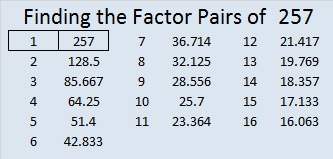# 257 and Level 5

• 257 is a prime number.
• Prime factorization: 257 is prime.
• The exponent of prime number 257 is 1. Adding 1 to that exponent we get (1 + 1) = 2. Therefore 257 has exactly 2 factors.
• Factors of 257: 1, 257
• Factor pairs: 257 = 1 x 257
• 257 has no square factors that allow its square root to be simplified. √257 ≈ 16.031How do we know that 257 is a prime number? If 257 were not a prime number, then it would be divisible by at least one prime number less than or equal to √257 ≈ 16.031. Since 257 cannot be divided evenly by 2, 3, 5, 7, 11, or 13, we know that 257 is a prime number.

257 is 2⁸ + 1 and is the second largest known Fermat Prime.  Click that link to see how unusual and cool Fermat Primes are!

Can you find the factors of the clues in this Level 5 puzzle?Print the puzzles or type the factors on this excel file: 12 Factors 2014-10-06